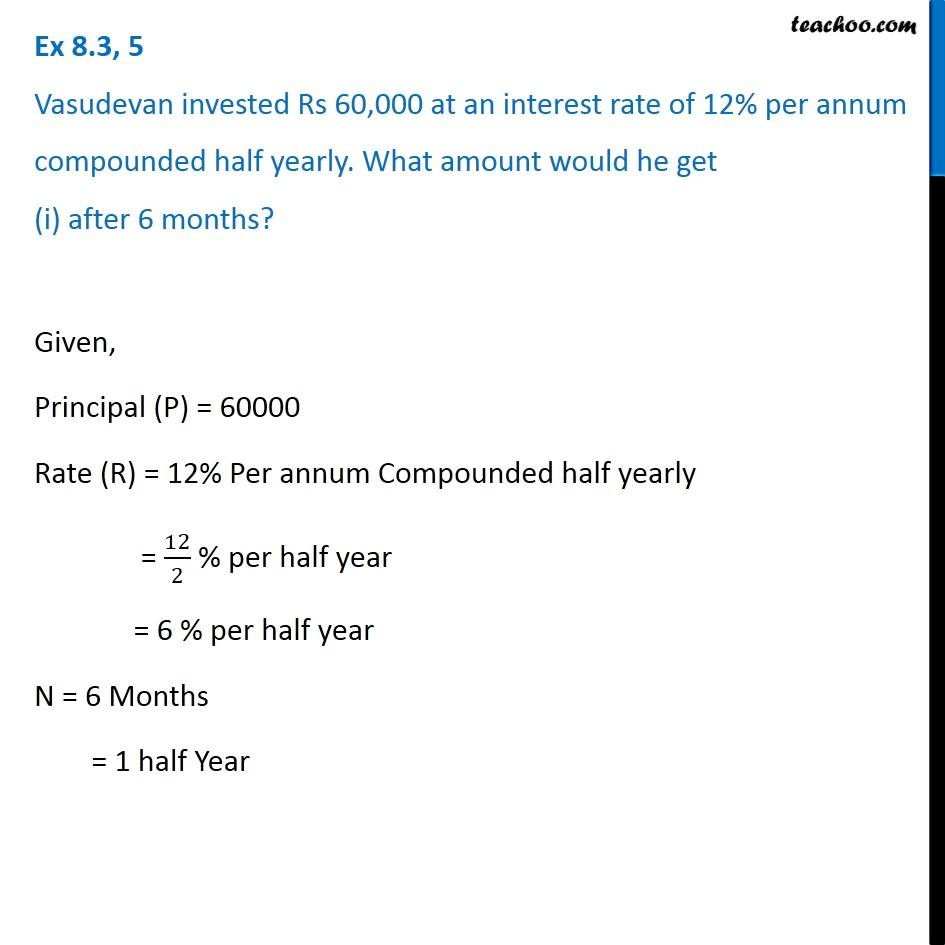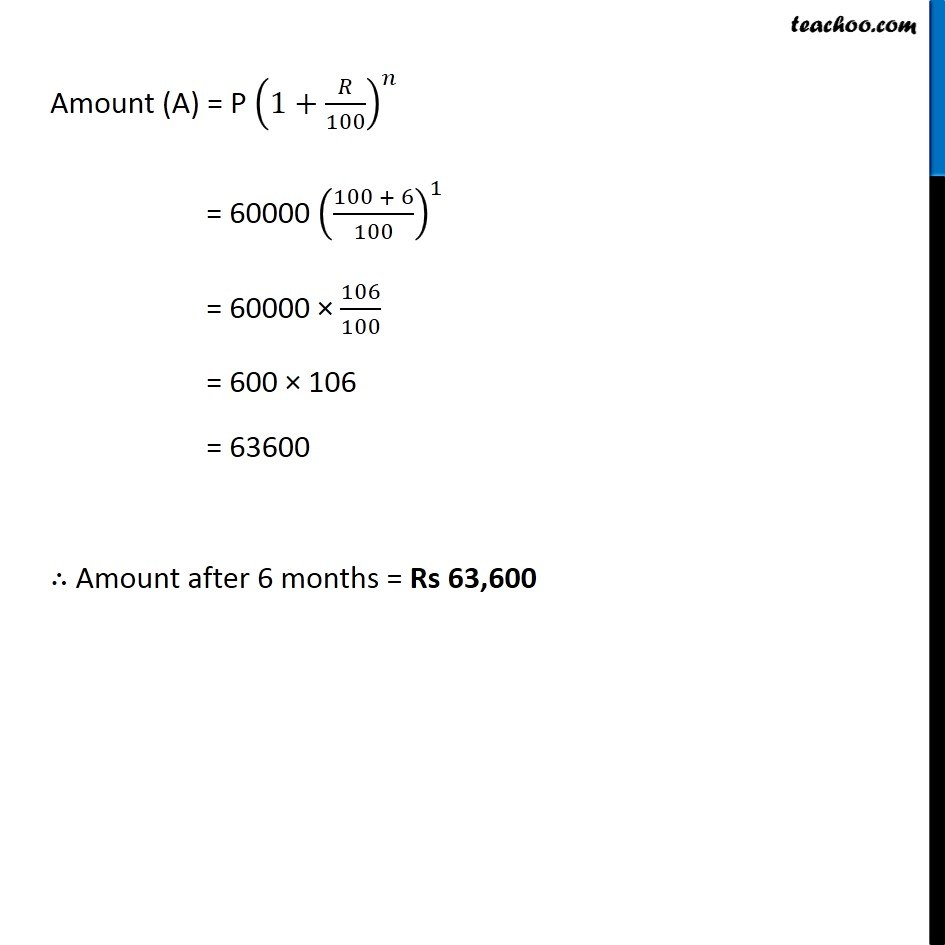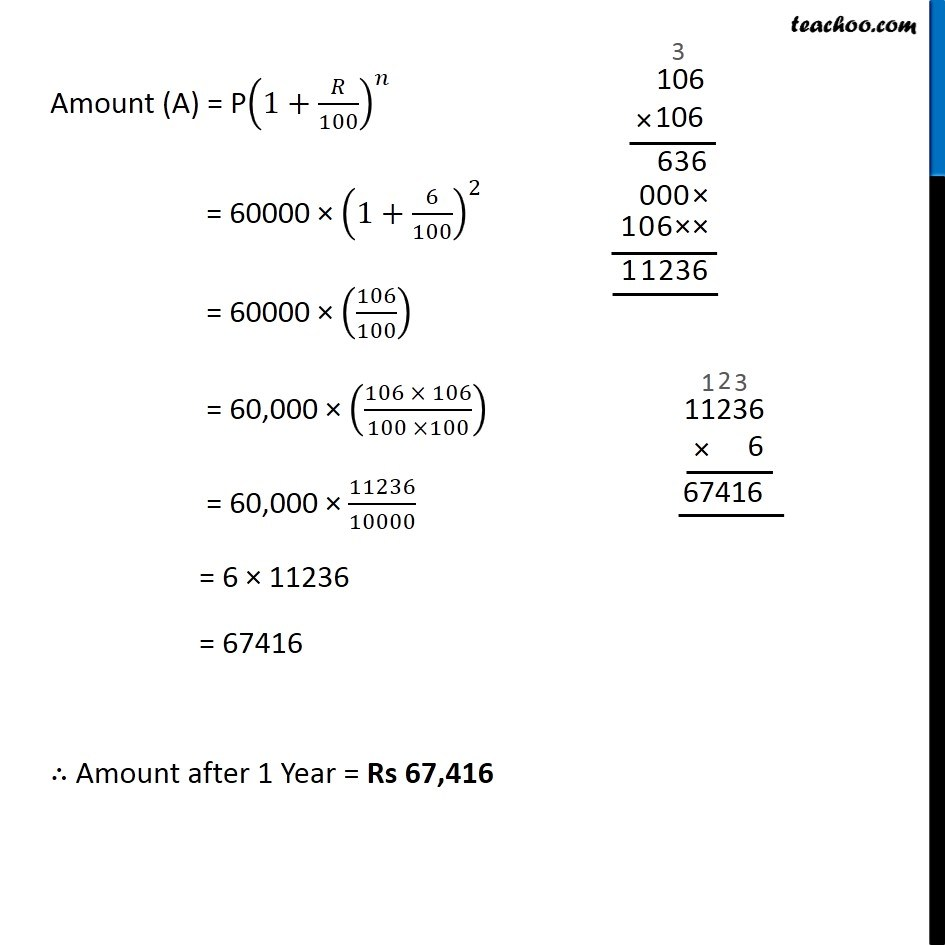Subscribe to our Youtube Channel - https://you.tube/teachoo

1. Chapter 8 Class 8 Comparing Quantities
2. Concept wise
3. Compound Interest compounded half yearly

Transcript

Ex 8.3, 5 Vasudevan invested Rs 60,000 at an interest rate of 12% per annum compounded half yearly. What amount would he get (i) after 6 months? Given, Principal (P) = 60000 Rate (R) = 12% Per annum Compounded half yearly = 12/2 % per half year = 6 % per half year N = 6 Months = 1 half Year Amount (A) = P (1+𝑅/100)^𝑛 = 60000 ((100 + 6)/100)^1 = 60000 × 106/100 = 600 × 106 = 63600 ∴ Amount after 6 months = Rs 63,600 Ex 8.3, 5 Vasudevan invested Rs 60,000 at an interest rate of 12% per annum compounded half yearly. What amount would he get (ii) after 1 year? Given, Principal (P) = 60000 Rate (R) = 12% Per annum Compounded half yearly = 12/2 % per half year = 6 % per half year n = 1 Year = 2 Half years Amount (A) = P(1+𝑅/100)^𝑛 = 60000 × (1+6/100)^2 = 60000 × (106/100) = 60,000 × ((106 × 106)/(100 ×100)) = 60,000 × 11236/10000 = 6 × 11236 = 67416 ∴ Amount after 1 Year = Rs 67,416

Compound Interest compounded half yearly